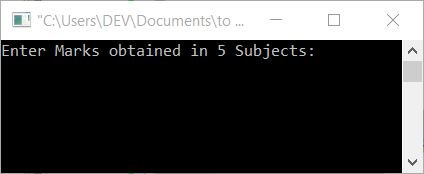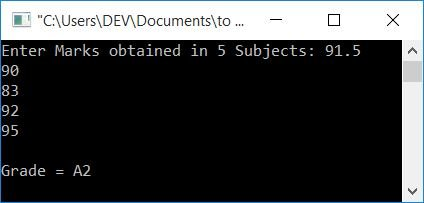# C Program to Calculate the Grade of a Student

In this article, you will learn and get code for finding the grade of a student on the basis of marks entered (in 5 subjects) by the user (at run-time). The grading system will be applied as shown in the table given below:

91-100 A1
81-90 A2
71-80 B1
61-70 B2
51-60 C1
41-50 C2
33-40 D
21-32 E1
0-20 E2

Here, the mark range is the average mark range. That is, if a student received 78, 74, 80, 72, and 76 in all five subjects, his or her average will be 76, and 76 falls between 71 and 80. So the grade will be B1.

Note: If the average mark of a student is 90.4, then his/her grade will be A2.

## Student Grade Program in C

The question is: write a program in C that asks the user to enter marks obtained in five subjects to calculate and print his or her grade based on the average marks. The answer to this question is given below:

```#include<stdio.h>
#include<conio.h>
int main()
{
int i;
float mark, sum=0, avg;
printf("Enter Marks obtained in 5 Subjects: ");
for(i=0; i<5; i++)
{
scanf("%f", &mark);
sum = sum+mark;
}
avg = sum/5;
if(avg>=91 && avg<=100)
printf("A1");
else if(avg>=81 && avg<91)
printf("A2");
else if(avg>=71 && avg<81)
printf("B1");
else if(avg>=61 && avg<71)
printf("B2");
else if(avg>=51 && avg<61)
printf("C1");
else if(avg>=41 && avg<51)
printf("C2");
else if(avg>=33 && avg<41)
printf("D");
else if(avg>=21 && avg<33)
printf("E1");
else if(avg>=0 && avg<21)
printf("E2");
else
printf("Invalid!");
getch();
return 0;
}```

This program is compiled and executed in the Code::Blocks IDE. Here is the snapshot of the sample run:Now supply any 5 inputs as marks for 5 subjects, say 91.5, 90, 83, 92, and 95, and press the ENTER key to see the output as given in the following snapshot:The above program finds the grade of a student using if-else statements.

Here is the modified version of the above program. In this program, we have allowed the user to enter the number of subjects and then to enter the marks obtained in all subjects as shown in the program given below:

```#include<stdio.h>
#include<conio.h>
int main()
{
int i, n;
float m, sum=0, a;
printf("How many Subject runs in your Institute ? ");
scanf("%d", &n);
printf("\nEnter marks obtained in %d Subjects: ", n);
for(i=0; i<n; i++)
{
scanf("%f", &m);
sum = sum+m;
}
a = sum/n;
if(a>=91 && a<=100)
printf("A1");
else if(a>=81 && a<91)
printf("A2");
else if(a>=71 && a<81)
printf("B1");
else if(a>=61 && a<71)
printf("B2");
else if(a>=51 && a<61)
printf("C1");
else if(a>=41 && a<51)
printf("C2");
else if(a>=33 && a<41)
printf("D");
else if(a>=21 && a<33)
printf("E1");
else if(a>=0 && a<21)
printf("E2");
else
printf("Invalid!");
getch();
return 0;
}```

The sample run of the above program is shown in the snapshot given below:#### The same program in different languages

C Quiz

« Previous Program Next Program »